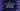# C++ STL accumulate method explanation with example## C++ STL accumulate() method explanation with example:

The accumulate method is used to get a result by accumulating all the values in a range. It uses an initial value and two ranges to accumulate all values. The std::accumulate adds all the element, or finds the sum of all the elements by default, but we can also define the operation to perform.

In this post, we will learn how to use the accumulate method with different examples.

### Syntax of accumulate:

The syntax of accumulate is as like below:

``T accumulate (InputIterator first, InputIterator last, T init, BinaryOperation op)``

Here,

• first is an input iterator to the initial position of a sequence.
• last is another input iterator to the final position of the sequence.
• init is the initial value of the final result.
• op is an optional value. It is the binary operation function. This function takes the current accumulator and the current element as its parameter.

### Return value of accumulate:

The accumulate function finds the sum of the values in the range [first, second) and init. It includes first but excludes second. If binary operation op is not given, it will use the operator +.

Let’s try accumulate with different example programs.

### Example 1: accumulate with an array of integers:

The following program uses accumulate with an array of integers:

``````#include <iostream>
#include <numeric>
using namespace std;

int main()
{
int givenArray[] = {1, 2, 3, 4, 5, 6, 7};

int sum = std::accumulate(givenArray, givenArray + 5, 0);

cout << "Sum: " << sum << endl;

return 0;
}``````

This will print:

``Sum: 15``

It takes 0 as the initial value and finds the sum from index 0 to index 4.

### Example 2: Example with a different start, end index and a different initial value:

Let’s try it with different start index, end index and initial value:

``````#include <iostream>
#include <numeric>
using namespace std;

int main()
{
int givenArray[] = {1, 2, 3, 4, 5, 6, 7};

int sum = std::accumulate(givenArray + 2, givenArray + 6, 4);

cout << "Sum: " << sum << endl;

return 0;
}``````

It will print:

``Sum: 22``

It calculates the sum of the numbers from index 2 to index 5 and adds 4 to it.

``4 + 3 + 4 + 5 + 6 = 22``

### Example 3: Example with vector:

Let’s try this method with a vector:

``````#include <iostream>
#include <numeric>
#include <vector>
using namespace std;

int main()
{
vector<int> v = {1, 2, 3, 4, 5, 6, 7};

int sum = accumulate(v.begin() + 2, v.begin() + 6, 10);

cout << "Sum: " << sum << endl;

return 0;
}``````

It will print:

``Sum: 28``

### Example 4: Example with the built-in binary predicate:

We can use a built-in binary predicate instead of +.

``````#include <iostream>
#include <numeric>
#include <functional>
using namespace std;

int main()
{
int givenArray[] = {1, 2, 3, 4, 5, 6, 7};

int sum = accumulate(givenArray, givenArray + 4, 1, multiplies<int>());

cout << "Sum: " << sum << endl;

return 0;
}``````

It will multiply all numbers from index 0 to index 3 with the initial value 1.

It will print:

``Sum: 24``

### Example 5: Custom predicate:

We can also pass a custom predicate as the last parameter. For example,

``````#include <iostream>
#include <numeric>
#include <functional>
using namespace std;

int customPredicate(int a, int n)
{
return a * n;
}

int main()
{
int givenArray[] = {1, 2, 3, 4, 5, 6, 7};

int sum = accumulate(givenArray, givenArray + 4, 1, customPredicate);

cout << "Sum: " << sum << endl;

return 0;
}``````

Here, customPredicate function is used as a custom predicate. It takes the accumulator and the current number as the parameters and returns the product of both.

It will give the same result.

``Sum: 24``